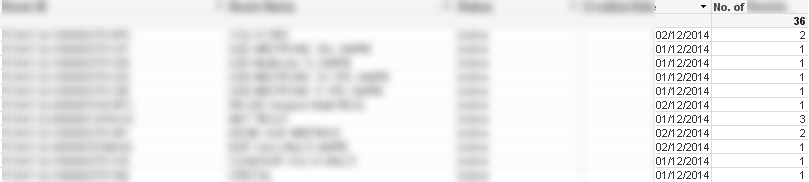# QlikView App Development

Discussion Board for collaboration related to QlikView App Development.

HighlightedNot applicable

## Display sum of rows from a chart, in a Text Object

Hello all,
I have a straight table with a few dimensions, and then an expression which I'm currently using as the last column which shows the sum of rec0rds, using "1 as recordCounter"
Using either of the two expressions:
=if(dimension <= vEndPeriod AND creationDateDiff >= vStartPeriod, recordCounter)
=sum(distinct{\$<dimension={'<=\$(vEndDate)'},dimension={'>=\$(vStartDate)'}>}recordCounter)
gives me the c0rrect total (21), shown below:However, I'd like to represent that total in a separate text object, and when I attempt to do this, I always end up with the number 36. This is due to some duplicate rec0rds in the data which is loaded over a number of days. If I use the above set expression but without the distinct, I get this 36:
=sum({\$<dimension={'<=\$(vEndDate)'},dimension={'>=\$(vStartDate)'}>}recordCounter)But if I try to use the distinct operat0r in the text object, I always end up with the value 1, no matter what combination of expressions/sum(if(xxxxx))/count(if(xxxxx)) I attempt.
How can I display the elusive 21 in a text object? 0r perhaps looking at it another way, how does qlikview calculate the "Sum of Rows" total mode in the straight table expression?
Many thanks, and excuse the use of zero instead of "o".  There's apparently a bug today that won't let you post a w0rd with o and r next to each other.
4 Replies
Honored Contributor II

Hi Andrew,

Try using

Regards

KC

Best Regards,
KC
Contributor II

## Re: Display sum of rows from a chart, in a Text Object

Here in this pivot table i m having the issue with the Performance Total it is varying .

It should be Sum([Coperate SLP])*[Performance])

Means (103*106)+(0*111)=109.18 but its displaying as 111 that is the problem over here.

Please Help me guys since a week i m striving to get it done but its not getting perfect.Partner

## Re: Display sum of rows from a chart, in a Text Object

hi

this a classic case for use of aggr function

it should look something like

sum({\$<dimension={'<=\$(vEndDate)'},dimension={'>=\$(vStartDate)'}>}

aggr(max({\$<dimension={'<=\$(vEndDate)'},dimension={'>=\$(vStartDate)'}>}recordCounter),yourdimension1,yourdimension2))Partner

## Re: Display sum of rows from a chart, in a Text Object

Try

Aggr(sum(distinct{\$<dimension={'<=\$(vEndDate)'},dimension={'>=\$(vStartDate)'}>}recordCounter),Dimension1)

You need to replace the Dimension1 part with a comma seperated list of the dimensions you use in your table